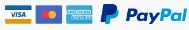100 worksheets, which helped my kids to learn addition and subtraction easily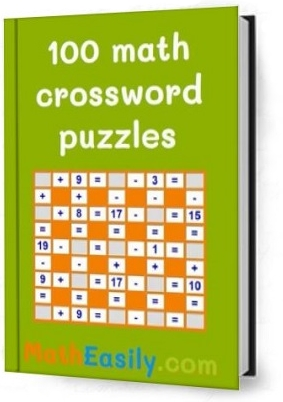Help your kids to learn addition and subtraction easily. Make math more enjoyable with these awesome math worksheets created on the basis of these online math crosswords.

US\$ 3.00

+/-

🙄

Are they making the same mistakes again and again?

📝

Or do you need to prepare your kids for a math exam?

This eBook of mixed addition and subtraction worksheets help kids to learn math in the easiest way. By filling crossword puzzles students review errors by themselves and so they learn basic math quickly.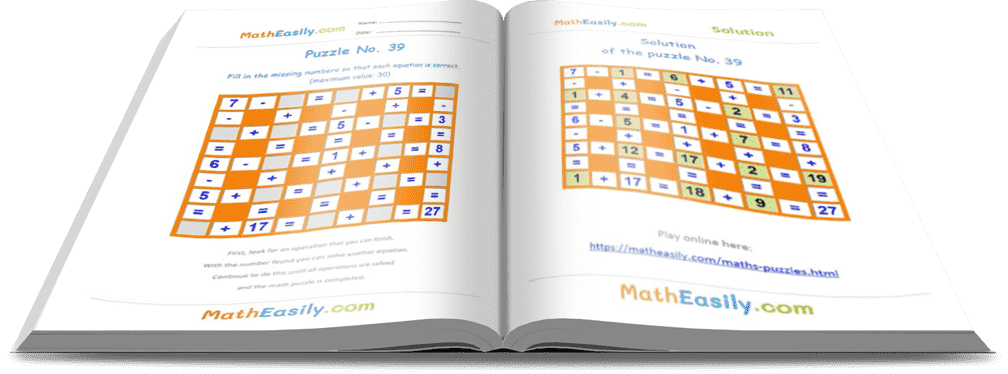Using different types of learning materials can really benefit students. Our math worksheets are both fun and challenging. Practice addition and subtraction facts by filling in the blanks for these equations.

## Which benefits does our eBook have for you?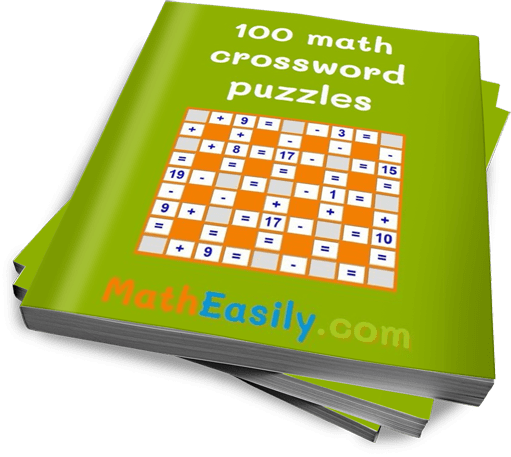Kids have a natural desire to learn new things - especially when they're having fun. Kids will enjoy our printable math puzzles and learn math at the same time.

You will save a lot of time because kids correct their errors by themselves. Kids love these puzzles, because they can learn math easily when they control their own learning.

No more tears and stress when learning math.

## What will you find inside?

Math crossword puzzles are a great way to learn the basic concepts of math. These puzzles are made for primary students. Worksheets are in PDF format, that you can print off.

This eBook contains 100 pages of crossword puzzles and 100 pages of their solutions. One page contains only the puzzle and the second page has the answer key.

100 pages of mixed addition and subtraction worksheets including:

• 25 pages to practice addition and subtraction up to 20
• 25 pages to practice addition and subtraction up to 30
• 25 pages to practice addition and subtraction up to 50
• 25 pages to practice addition and subtraction up to 100

The missing numbers are in different places for each worksheet.

The ebook contains 100 pages of answers (solutions) also.

How to solve the puzzle? First, look for an operation that you can finish. With the number found you can solve another equation. Continue to do this until all operations are solved and the math puzzle is completed.

Kids can check their answer with the given solution.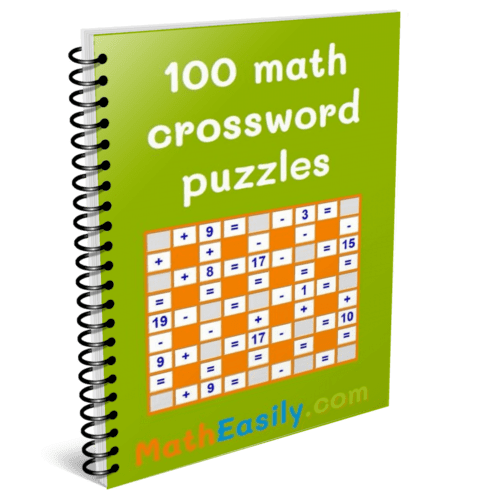So if you are a parent or a teacher, math puzzles are a great tool for helping kids to master math.

Ebook includes 100 printable worksheets in PDF. Each worksheet consists of two pages. The first page contains only the crossword puzzle, while the second page has also the answers.

• 100 pages to practice addition and subtraction
• 100 pages of solutions
• a lot of joy

US\$ 3.00